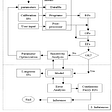# What is multicollinearity?

Multicollinearity is defined as a condition where two or more explanatory variables are related amongst themselves which may cause misleading predictions.

# When is multicollinearity an issue?

Multicollinearity is an issue when the correlations between the columns may change with change in the conditions.

For example let us take the scenario of the stock market before and after the COVID-19 pandemic.

Before the pandemic, the automobile sector and pharmaceutical sector were both doing well in their respective fields, which also implies high correlation.

If we calibrate a machine learning model based on pre COVID scenario and deploy that machine learning model without checking for multicollinearity, initially it may give accurate results

But after COVID pandemic, we have observed that the automobile sector has seen a decline in it’s performance and the pharmaceutical sector has seen an increase in it’s performance.

Now the predictions given by our machine learning model which was calibrated before the COVID-19 pandemic using the correlation between the sectors at that time will give us inaccurate predictions hence we will need to calibrate a new model

To avoid the multicollinearity trap, we should not include highly correlated explanatory variables and try to adhere to the assumption of independence of regressors of the Gauss Markov setup

# Steps to detect multicollinearity

We can detect multicollinearity by the following ways

1. If we have domain knowledge about the dataset we are working with, we can judge the highly correlated variables by the domain knowledge for example we know that in the case of predicting house prices, carpet area and plot area are highly correlated variables and we can drop one of them accordingly
2. We can look at the correlation matrix or correlation heatmap and look out for high values in the dataset
3. We can look at the variance inflation factor (discussed later in this article) of each column and look out for values of variance inflation factor greater than 5

# Variance Inflation Factor (VIF)

VIF is a metric which is used for measuring the amount of multicollinearity in the dataset by regressing an explanatory variable on other explanatory variables and then looking at the R squared of that model

Let the model be

Here X1 can be expressed as a linear combination of all other regressors excluding X1

And R1 squared will be the R squared of this model, similarly VIF of X1 will be

The value of VIF will increase if the value of R1 squared will increase, which signifies that X1 can be efficiently expressed as a linear combination of other regressors implying that X1 can be explained by other regressors and X1 should be dropped

Similarly, we calculate VIF for all the regressors and the regressors having VIF greater than 5 should be dropped as they have high multicollinearity.

# Steps for solving Multicollinearity

1. Drop variables on the basis of domain knowledge if possible
2. Look out for variables with high correlation coefficient in the correlation matrix and drop them
3. Look out for variables with a VIF value greater than 5 and drop them
4. Use lasso regression as the regularization function in lasso regression automatically drops one variable
5. Use PCA technique to solve the issue of multicollinearity

--

--

--

A data science enthusiast currently doing bachelor's degree in data science

Love podcasts or audiobooks? Learn on the go with our new app.

## How to Track Indicators for Inflation## New Maps and Charts Showing Power of Data Visualization — DataViz Weekly## Sanitary Sewer Model Calibration Review## Do University Towns in US have their mean housing prices less effected during recession?## Best times to Visit Boston: Analyzing Pricing and Availability of Airbnb Units## Premier League Data Analysis## How to become a Data Scientist ? Skills required ?## Great Expectations — The Good and the Bad## Aayushmaan Jain

A data science enthusiast currently doing bachelor's degree in data science

## How Data Science is helping to understand brain intricacies:## Basics understanding of Regression in Machine Learning## What’s Machine Learning?